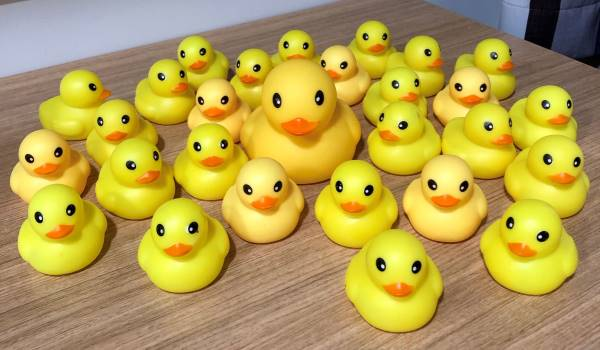Training SiteInput: Standard Input (stdin)
Output: Standard Output (stdout)
Memory limit: 128 megabytes
Time limit: 1.0 seconds

You may remember from Round 2 that Thomas really loves ducks. (Don't worry if you haven't done Round 2, though)You may have wondered how Thomas got all those ducks. The answer is that he buys them all in bulk from his favorite e-commerce website, AliDirect™. Specifically, he can buy ducks in lots of 2 for $A$ dollars, and lots of 3 for $B$ dollars.

Thomas wants to buy exactly $N$ ducks for the upcoming January training camp. Help him find the minimum amount he has to pay.

## Input

The first line contains a single integer, $N$, the number of ducks Thomas would like to buy.

The second line contains a single integer, $A$, the cost of buying one lot of 2 ducks.

The third line contains a single integer, $B$, the cost of buying one lot of 3 ducks.

## Output

You should print a single integer – the minimum cost of buying exactly $N$ ducks.

It is guaranteed that it will always be possible to buy exactly $N$ ducks.

## Constraints

• $2 \le N \le 100\,000$ (Thomas buys a lot of ducks)
• $1 \le A,B \le 100$

• Subtask 1 (+20%): $A = 2, B = 3$
• Subtask 2 (+30%): $A = 3, B = 5$
• Subtask 3 (+50%): No further restrictions apply

## Sample Explanations

### Sample Input 1

The only way to buy 4 ducks is to buy 2 lots of 2 ducks, for a total of $2 \times 2 = 4$ dollars.

### Sample Input 2

There are two different ways to buy 11 ducks:

• The first way is to buy 4 lots of 2 ducks and 1 lot of 3 ducks for a total of $4 \times 3 + 1 \times 5 = 17$ dollars.
• The second way is to buy 1 lot of 2 ducks and 3 lots of 3 ducks for a total of $1 \times 3 + 3 \times 5 = 18$ dollars.

The first way is cheaper, so the answer is 17.

• ### Sample Input 1

4
2
3


### Sample Output 1

4

• ### Sample Input 2

11
3
5


### Sample Output 2

17